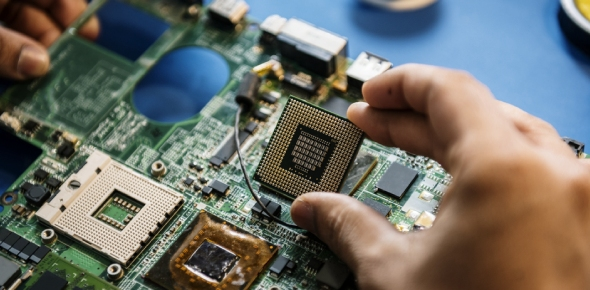# Microprocessor & Microcontroller (Cs502)

40 Questions | Attempts: 696
ShareSettings• 1.
Which is the microprocessor comprises:
• A.

Register section

• B.

ALU

• C.

Control unit

• D.

All of these

• 2.
What will be stored by register?
• A.

Data

• B.

Operands

• C.

Memory

• D.

None of these

• 3.
Accumulator based microprocessor example are:
• A.

Intel 8085

• B.

Motorola 6809

• C.

A and B

• D.

None of these

• 4.
A sets of registers can contain:
• A.

Data

• B.

• C.

Result

• D.

All of these

• 5.
There are primarily two types of register:
• A.

General purpose register

• B.

Dedicated register

• C.

A and B

• D.

None of these

• 6.
BCD stands for:
• A.

Binary coded decimal

• B.

Binary coded decoded

• C.

Both a & b

• D.

None of these

• 7.
Which is used to store critical pieces of data during subroutines and interrupts:
• A.

Stack

• B.

Queue

• C.

Accumulator

• D.

Data register

• 8.
The process by which data will be submitted in the stack is:
• A.

Push

• B.

Enter

• C.

Submit

• D.

None of these

• 9.
Each memory location has:
• A.

• B.

Contents

• C.

Both A and B

• D.

None of these

• 10.
IP stands for:
• A.

Instruction pointer

• B.

Instruction purpose

• C.

Instruction paints

• D.

None of these

• 11.
The size of data bus in 8085 is:
• A.

4 bit

• B.

6 bit

• C.

8bit

• D.

16 bit

• 12.
• A.

8 bit

• B.

16 bit

• C.

24 bit

• D.

32 bit

• 13.
Data bus is:
• A.

Uni directional

• B.

Bi directional

• C.

Both A and B

• D.

None of these

• 14.
• A.

Bi directional

• B.

Uni directional

• C.

Both A and B

• D.

None of these

• 15.
In 8085, all the results are stored in:
• A.

Accumulator

• B.

Stack Pointer

• C.

Program Counter

• D.

Instruction Pointer

• 16.
In 8085, register pairs are:
• A.

AB, CD, EF, HM

• B.

BC, DE, HL

• C.

All of the above

• D.

None of these

• 17.
When 9th bit will come, after any arithmetic operation, carry flag will be:
• A.

Set

• B.

Reset

• C.

All of the above

• D.

None of the above.

• 18.
In an arithmetic result, if even number of ones are present, which flag will be set?
• A.

Carry

• B.

Auxiliary Carry

• C.

Zero

• D.

Parity

• 19.
Auxiliary carry will be set when:
• A.

Carry will be passed from bit 0 to bit 1

• B.

Carry will be passed from bit 3 to bit 4

• C.

All of the above

• D.

None of these

• 20.
Z flag will be set when:
• A.

Final result is all ones

• B.

Final result is all zeros

• C.

All of the above

• D.

None of the above

• 21.
• A.

Opcode, Operand and Process

• B.

Operand, Opcode and Process

• C.

All of the above

• D.

None of these

• 22.
LDA 2050 - example of
• A.

Immediate mode

• B.

Indirect mode

• C.

Direct mode

• D.

Implicit mode

• 23.
Example of immediate addressing mode is:
• A.

LDA 2050

• B.

MOV A,B

• C.

MVI H, 23

• D.

STAX B

• 24.
Indirect register addressing mode is explained by:
• A.

MOV A,M

• B.

MOV A,B

• C.

MVI A,09

• D.

None of these

• 25.
MOV H,L stands for
• A.

Content of H is going to L

• B.

Content of L is coming to H

• C.

All of the above

• D.

None of the above

## Related TopicsBack to top
×

Wait!
Here's an interesting quiz for you.